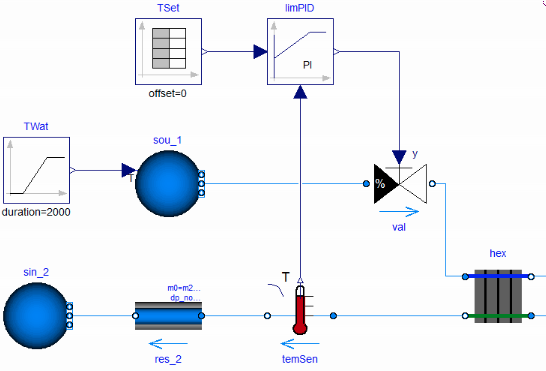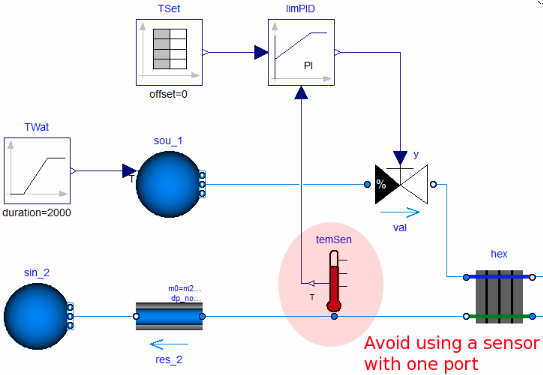#.Annex60.Fluid.Sensors.UsersGuide

## Information

This package contains models of sensors. There are models with one and with two fluid ports.

#### Selection and parameterization of sensor models

When selecting a sensor model, a distinction needs to be made whether the measured quantity depends on the direction of the flow or not, and whether the sensor output signal is the product of the mass flow rate and a medium property.

Output signals that depend on the flow direction and are not multiplied by the mass flow rate are temperature, relative humidity, water vapor concentration X, trace substances C and density. For such quantities, sensors with two fluid ports need to be used. An exception is if the quantity is measured directly in a fluid volume, such as modeled in models of the package Annex60.Fluid.MixingVolumes. Therefore, to measure for example the outlet temperature of a heat exchanger, the configuration on the left in the figure below should be used, and not the configuration on the right. For an explanation, see Modelica.Fluid.Examples.Explanatory.MeasuringTemperature.

Correct useExcept for the mass flow rate sensor, all sensors with two ports can be configured as dynamic sensors or as steady-state sensor. For numerical reasons, if the sensor output signal is not multiplied by the mass flow rate, then it is strongly suggested to configure these sensors as a dynamic sensor, which the default setting. Configuring a sensor as a dynamic sensor is done by setting the time constant to a non-zero value. Typically, setting `tau=10` seconds yields good results. For `tau=0`, numerical problems may occur if mass flow rates are close to zero.

If the sensor output signal is the product of mass flow rate times a measured fluid property, such as sensors for volumentric flow rate or enthalpy flow rate, then the sensor is by default configured as steady-state sensor. These sensors may be configured by the user as a dynamic sensor by setting `tau > 0`, but there is typically little benefit as these sensors typically do not cause numerical problems. The reason is that these sensors multiply the quantity that is carried by the flow, such as specific enthalpy h by the mass flow rate to output the measured signal Ḣ=ṁ h. Hence, as the mass flow rate goes to zero, the sensor output signal also goes to zero, which seems to avoid numerical problems.

For static pressure measurements, sensors with one or with two ports can be used for all connection topologies.

The table below summarizes the recommendations for the use of sensors.

Measured quantity One port sensor Two port sensor
steady-state (`tau=0`) dynamic (`tau > 0`)
temperature
relative humidity
mass fraction
trace substances
specific enthalpy
specific entropy
use only if connected to a volume avoid recommended
volume flow rate
enthalpy flow rate
entropy flow rate
- recommended recommended
pressure recommended recommended recommended

#### Sensor Dynamics

##### Dynamic response to fluid flowing through the sensor

If a sensor is configured as a dynamic sensor by setting `tau > 0`, then the measured quantity, say the temperature T, is computed as

τ   dT ⁄ dt = |ṁ| ⁄ ṁ0   (θ-T),

where τ is a user-defined time constant of the sensor (a suggested value is around 10 seconds, which is the default setting for the components), dT ⁄ dt is the time derivative of the sensor output signal, |ṁ| is the absolute value of the mass flow rate, 0 is the user-specified nominal value of the mass flow rate and θ is the temperature of the medium inside the sensor. An equivalent physical model of such a sensor would be a perfectly mixed volume with a sensor that outputs the temperature of this volume. In this situation, the size of the volume would be V=τ   ṁ0 ⁄ ρ, where ρ is the density of the fluid.

##### Dynamic response to ambient temperature

For the sensor Annex60.Fluid.Sensors.TemperatureTwoPort, by setting `transferHeat = true`, heat transfer to a fixed ambient can be approximated. The heat transfer is computed as

τHeaTra   dT ⁄ dt = (TAmb-T),

where τHeaTra is a fixed time constant and TAmb is a fixed ambient temperature. Setting `transferHeat = true` is useful if the sensor output T is used to switch the mass flow rate on again. If `transferHeat = false`, then the sensor output T remains constant if the mass flow rate is zero and hence a fan or pump controller that uses this signal may never switch the device on again. If the sensor output T is not used to switch on the mass flow rate, then in general one can use `transferHeat=false`.

Note that since in practice the heat transfer is due to a combination of ambient temperature and upstream or downstream fluid temperature, for example by two-way buoyancy-driven flow inside the duct or pipe, the model uses as an approximation a fixed ambient temperature. Since the sensor is not affecting the temperature of the medium, this approximation of the heat transfer does not add or remove heat from the fluid.

##### Combined dynamic response

For the sensor Annex60.Fluid.Sensors.TemperatureTwoPort, if both dynamic effects are enabled, then the output T is computed as

dT ⁄ dt = |ṁ| ⁄ ṁ0   (θ-T) ⁄ τ + (TAmb-T) ⁄ τHeaTra.

#### Implementation

The above equation is implemented in such a way that it is differentiable in the mass flow rate.

Note that the implementation of the dynamic sensors does not use the model Annex60.Fluid.MixingVolumes. The reason is that depending on the selected medium model, the mixing volume may introduce states for the pressure, species concentration, trace substance, specific enthalpy and specific entropy. Not all states are typically needed to model the dynamics of a sensor. Moreover, in many building system applications, the sensor dynamics is not of concern, but is rather used here to avoid numerical problems that steady-state models of sensors cause when flow rates are very close to zero.

Generated at 2020-04-01T01:39:02Z by OpenModelicaOpenModelica 1.16.0~dev-259-g8a5138f using GenerateDoc.mos